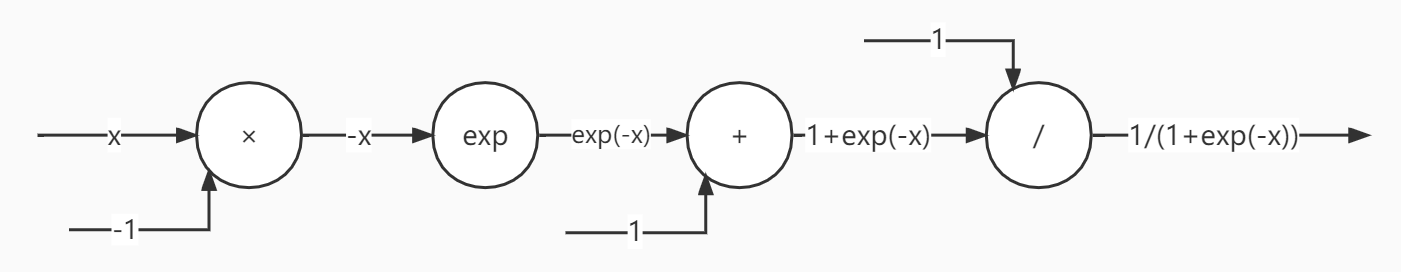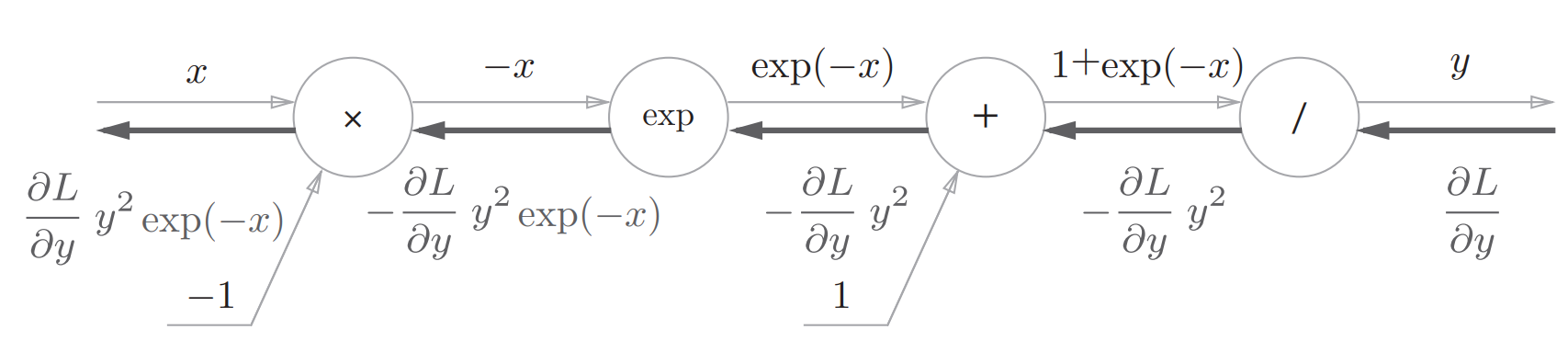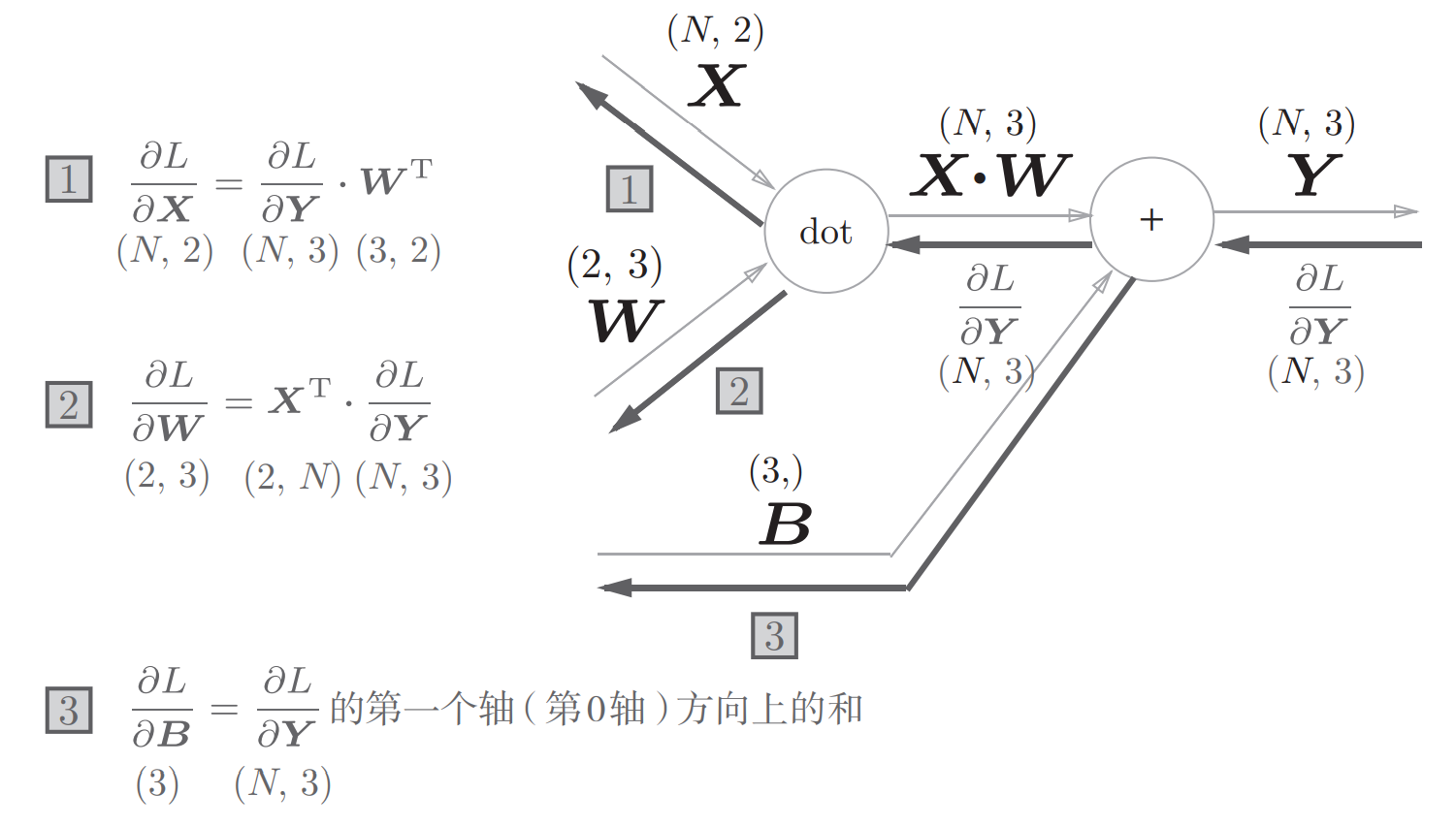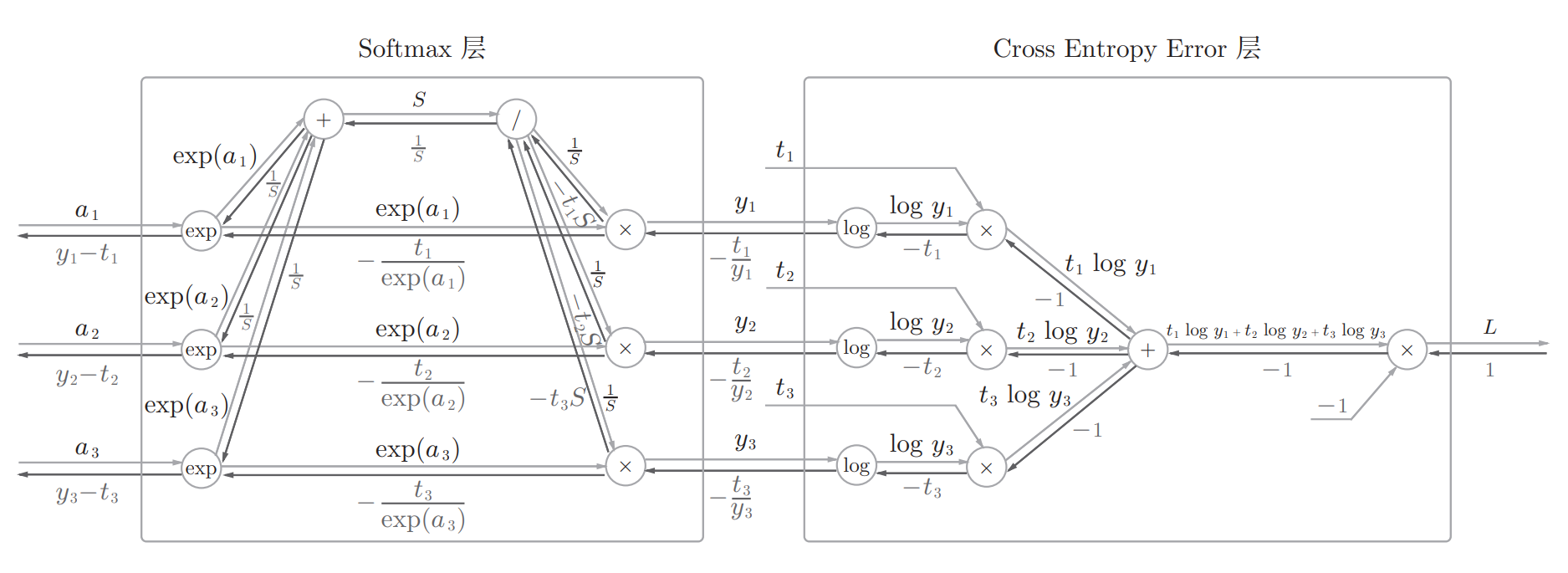# 【深度学习笔记】误差反向传播（2）

【深度学习笔记】误差反向传播（1）中，我们了解了一些误差反向传播的知识，现在，我们就可以把理论转化成代码，去设计实现一个带有反向传播的神经网络，接下来我们一起实现一下反向传播。

## 加法层和乘法层

### 加法层

class AddLayer:
def __init__(self):
pass

def forward(self, x, y):
out = x + y
return out

def backward(self, dout):
dx = dout * 1
dy = dout * 1
return dx, dy

### 乘法层

class MulLayer:
def __init__(self):
self.x = None
self.y = None

def forward(self, x, y):
self.x = x
self.y = y
out = x * y
return out

def backward(self, dout):
dx = dout * self.y
dy = dout * self.x
return dx, dy

## 神经网络中各层的反向传播实现

### 激活函数层

#### ReLU函数：

$$y=\begin{cases} x & \text{ if } x>0 \\ 0 & \text{ if } x\leqslant 0 \end{cases}$$

$$\frac{\partial y}{\partial x}=\begin{cases} 1 & \text{ if } x>0 \\ 0 & \text{ if } x\leqslant 0 \end{cases}$$

class Relu:
def __init__(self):

def forward(self, x):
out = x.copy()
return out

def backward(self, dout):
dx = dout
return dx

#### Sigmoid函数：

$$y=\frac{1}{1+e^{-x}}$$$$\frac{\partial L}{\partial y}y^2e^{-x}=\frac{\partial L}{\partial y}\frac{1}{(1+e^{-x})^2}e^{-x} \\ =\frac{\partial L}{\partial y}\frac{1}{1+e^{-x}} \frac{e^{-x}}{1+e^{-x}} \\ =\frac{\partial L}{\partial y}y(1-y)$$

class Sigmoid:
def __init__(self):
self.out = None

def forward(self, x):
out = 1 / (1 + np.exp(-x))
self.out = out
return out

def backward(self, dout):
dx = dout * (1.0 - self.out) * self.out
return dx

## Affine层class Affine:
def __init__(self, W, b):
self.W = W
self.b = b
self.x = None
self.dW = None
self.db = None

def forward(self, x):
self.x = x
out = np.dot(x, self.W) + self.b
return out

def backward(self, dout):
dx = np.dot(dout, self.W.T)
self.dW = np.dot(self.x.T, dout)
self.db = np.sum(dout, axis=0)
return dx

## Softmax-With-Loss层

Softmax函数会将输入值正规化之后再输出，比如在一个二分类的神经网络中，最后一层输出的结果为9.1, 0.9，那么softmax函数会将这两个结果进行正规化为0.91, 0,09，即属于第一类的可能是91%，属于第二类的可能是9%。class SoftmaxWithLoss:
def __init__(self):
self.loss = None # 损失
self.y = None # softmax的输出
self.t = None # 监督数据（one-hot vector）

def forward(self, x, t):
self.t = t
self.y = softmax(x)
self.loss = cross_entropy_error(self.y, self.t)
return self.loss

def backward(self, dout=1):
batch_size = self.t.shape
dx = (self.y - self.t) / batch_size
return dx